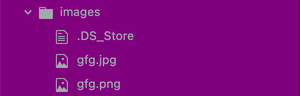# How to convert JPG to PNG using Node.js ?

• Last Updated : 06 Apr, 2021

JPG and PNG both are widely used image formats. Using these formats allows the user to contain and use digital images. In this article, we will discuss how to convert a given JPG image to a PNG image using Node.js which can then be helpful to use the image in our web application.

Jimp (JavaScript Image Manipulation Program):

Jimp is an image processing library that is written entirely in JavaScript and is used by Node. For the conversion of JPG to PNG, we will be using Jimp.

Installation:

To install Jimp in our project, we simply have to install its npm module into our project. This can be done by executing the following line in our terminal.

`npm install --save jimp`

Usage:

To use it in our project, we will need to import the package into our index.js file by using the following line of code.

`const Jimp = require("jimp");`

Now that we have Jimp installed and ready to use, we can move ahead to convert JPG to PNG.

JPG to PNG Conversion:

We will use .read() and .write() method to do the conversion. Let’s consider an example where we will have an input JPG image and then convert it into PNG image.

Example:

Let’s suppose we have a JPG image given below as input.gfg.jpg

We are keeping the code in the images folder. Now to convert it into PNG format, our script code will look like the following.

## index.js

 `// Importing the jimp module``const Jimp= require(``"jimp"``);`` ` `//We will first read the JPG image using read() method. ``Jimp.read(``"images/gfg.jpg"``, ``function` `(err, image) {``  ``//If there is an error in reading the image, ``  ``//we will print the error in our terminal``  ``if` `(err) {``    ``console.log(err)``  ``} ``  ``//Otherwise we convert the image into PNG format ``  ``//and save it inside images folder using write() method.``  ``else` `{``    ``image.write(``"images/gfg.png"``)``  ``}``})`

On executing the code using node, we should be able to get the PNG converted image in our images folder as shown below.

Output:My Personal Notes arrow_drop_up